# Amplitude Modulation (AM)

## Overview

Modulation is the process of varying the properties of a periodic waveform in order to represent analogue or digital information. The periodic waveform used is called a carrier wave, and usually has a sinusoidal waveform. The property that is varied by the modulation process depends on the kind of modulation scheme being used. It can be the amplitude, frequency, or phase of the carrier wave, or even some combination thereof.

The underlying reason for wanting to modulate an information-carrying signal onto a carrier wave in the first place is that the signal is unsuitable for the transmission medium over which we want to send it. An example that most people will be familiar with is broadcast radio, which typically broadcasts music, news, weather forecasts and so on. The frequencies we actually hear when we listen to a radio broadcast belong to the audio spectrum, which ranges from about 20 Hz at the lower end up to around to 20 kHz at the upper limit of hearing.

Sending audio-frequency signals over a wired transmission line is not a problem, although the public switched telephone system limits the frequencies used to between 300 and 3,400 hertz, giving a total bandwidth of 3,100 hertz. This is perfectly adequate for purely voice transmission, since the higher frequencies in the human voice (i.e. those above 3,400 hertz) are not really needed for recognisable speech reproduction. The use of a limited bandwidth also makes the telephone system much simpler to implement and maintain from an engineering perspective.

Sending audio signals wirelessly using radio frequencies is not so easy. The audio spectrum spans the extremely low frequency (ELF), super low frequency (SLF), ultra low frequency (ULF), and very low frequency (VLF) bands, which cover the range from 3 Hz up to 30 kHz. Apart from the fact that most of these frequencies are used for highly specialised military applications, there is another more serious problem. It turns out that the size of the antenna needed to transmit radio waves is inversely proportional to the frequency of those radio waves; the antennae needed to transmit at these frequencies can vary in length from a few kilometres up to several tens of kilometres!

In order to broadcast audio signals in a cost-effective and efficient manner, we need to use higher frequencies. There are a few AM radio stations that use the low frequency (LF) or longwave band, typically broadcasting on frequencies ranging from 150 kHz up to around 280 kHz, although the transmitters still need fairly large antennae - AM radio stations that broadcast on the longwave band typically use mast antennae with heights of around 150 metres. Most AM radio stations use the medium frequency (MF) band which occupies frequencies from 300 kHz up to 3 MHz.

The frequencies in the low and medium frequency bands are obviously much higher than the frequencies in the audio band, so in order to get a high frequency signal to "carry" a low frequency signal, we have to use some form of modulation. In the case of AM radio, the amplitude of a radio-frequency carrier wave is varied in proportion to that of the much lower frequency audio signal. We can consider amplitude modulation to be a form of analogue modulation because the modulating signal is a continuously time-varying signal that directly represents the information we want to transmit - in other words, an analogue signal.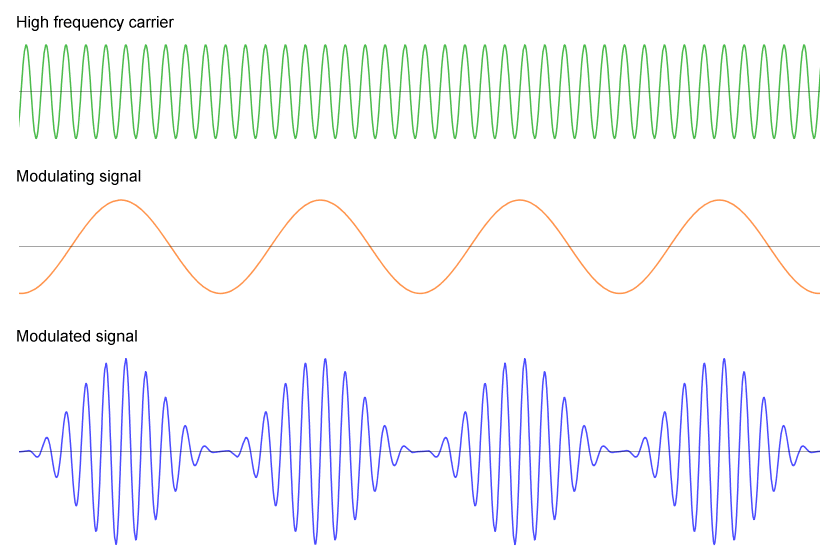Amplitude modulation - a low-frequency signal is used to modulate a high-frequency carrier

A simple form of amplitude modulation was originally used to modulate audio voice signals onto a low-voltage direct current (dc) carrier on a telephone circuit. A microphone in the telephone handset acts as a transducer, and uses the sound waves produced by the human voice to vary the current passing through the circuit. At the other end of the telephone line, a second transducer (in the form of a small loudspeaker mounted in the remote handset) uses the varying voltage to produce sound waves that are close enough to the original speech patterns to be recognisable as the voice of the caller.

## Basic amplitude modulation

To produce an amplitude modulated signal, the instantaneous amplitude of a radio frequency carrier wave is varied in direct proportion to that of the modulating signal. The modulating signal carries the required information and often consists of audio data, as in the case of AM radio broadcasts. The carrier is modulated by adding the modulating signal to it in a mixer. A simplified AM radio transmitter system is shown below.A simplified AM radio transmitter system

Amplitude modulating a carrier wave involves adding two different signals - the high-frequency carrier itself, which is generated by an oscillator, and the modulating signal, usually an audio signal. The result of mixing the two signals is a third signal consisting of the carrier signal and two sidebands (we'll enlarge on the topic of sidebands in due course). If we consider these signals in the time domain (i.e. with respect to time t in seconds), the carrier signal c(t) is:

c(t)  =    Ac  cos(2πfc t)

and the modulating signal is:

m(t)  =    Am  cos(2πfm t)

where Ac  and Am  are the amplitudes of the carrier signal and the modulating signal respectively, and fc  and fm  are the respective frequencies of those signals. The resulting amplitude modulated signal s(t) is therefore:

s(t)  =  [Ac  + Am  cos(2πfm t)] cos(2πfc t)

## Heterodyning

Modulating a carrier wave by adding another, much lower frequency signal results in a signal that has most of its power concentrated in the carrier, with the rest shared between two sidebands, one above the carrier in frequency and one below it. The highest frequency in the modulating signal is typically significantly less than ten percent of that of the carrier (AM radio usually places an upper limit of limits of 5 kHz on the frequencies used to modulate the carrier).

The process of creating sideband frequencies by adding another signal to the carrier is known as heterodyning. In the simplest case, the carrier is modulated by adding a single-frequency sine wave signal to it, changing the carrier's shape (sometimes called its envelope) as illustrated below. The sideband frequencies account for approximately 33% of the transmitted power. If a more complex modulating signal (such as an audio signal) is used to modulate the carrier, the sidebands account for only about 20-25% of the total transmitted power.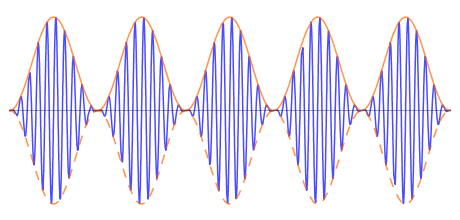The amplitude modulation process shapes the carrier's envelope

Consider a 100 kHz carrier that is modulated by a steady audio signal (or tone) of 5 kHz. When these signals are added, two sidebands are produced. One sideband has a frequency equal to the sum of the carrier and the modulating signal (100 kHz + 5 kHz = 105 kHz), while the other sideband has a frequency equal to the difference between the carrier and the modulating signal (100 kHz - 5 kHz = 95 kHz). The two sidebands are 5 kHz equidistant from the carrier (one above it and one below it), giving a total bandwidth for the modulated signal of 10 kHz (105 kHz - 95 kHz). The resulting frequency spectrum is illustrated below.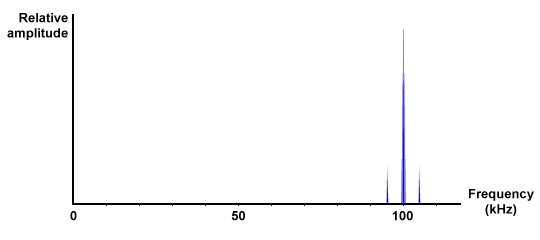A 100 kHz carrier modulated by a 5kHz audio tone

Most audio signals are more complex than a single-frequency audio tone, and are composed of many different frequencies. When a carrier is modulated with a complex audio signal, all of the frequencies present in the audio signal are represented in the resulting output signal, the total bandwidth of which is the difference between the sum and difference values of the carrier and the highest frequency component of the modulating signal.

In a nutshell, the bandwidth of the output signal will be twice that of the input signal. If the input signal contains frequency components ranging from 0 - 6 kHz, using a 100 kHz carrier results in output frequencies of between 94 kHz and 106 kHz – a bandwidth of 12 kHz. This produces a more complex frequency spectrum, which might look something like the one shown below.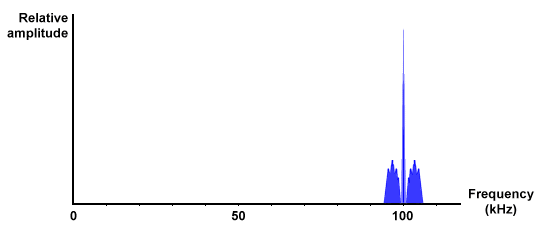A 100 kHz carrier modulated by an audio signal containing frequencies of up to 6 kHz

## Superheterodyning

The carrier frequencies used in some applications are very high (radar, for example, can use frequencies of up to 300 GHz). At very high frequencies, many standard electronic components won't function properly. A superheterodyne receiver reduces the frequency of an incoming signal by adding another frequency to it using a mixer prior to processing (a process known as superheterodyning). The centre frequency of the resulting signal is called the intermediate frequency (or beat frequency), and is equal to the difference between the carrier frequency and that of the local oscillator.

The receiver uses a tuner to select the required carrier frequency and filter out unwanted signals. The local oscillator is linked to the tuner to ensure that any changes in carrier frequency results in a corresponding change in the local oscillator's frequency, thus ensuring that the intermediate frequency will always have the same value. This both simplifies the design of the receiver and reduces its cost, because most of its components are only required to operate at the fixed intermediate frequency rather than over a range of frequencies. A simple superheterodyne receiver system is shown below.The band-pass filter in the tuner filters out all signals except the selected carrier frequency and its sidebands. The receiver bandwidth is usually some fraction of the carrier frequency. A receiver bandwidth of 2%, for example, means that all signals between 2% above and 2% below the carrier frequency are allowed to pass through the filter.

For a carrier frequency of 850 kHz, this would mean that all signals between 833 kHz and 867 kHz are accepted by the receiver. If the same fraction is applied to the intermediate frequency, then for a fixed intermediate frequency of 452 kHz, only signals that are within the range 443 kHz to 461 kHz will pass. The local oscillator is set to 398 kHz to reduce the 850 kHz carrier to the 452 kHz intermediate frequency (850 kHz - 398 kHz = 452 kHz).

Any adjacent signals are also superheterodyned, but remain at the same margin above and below the original signal. If the incoming signal includes interference at 863 kHz, a conventional 2% receiver will allow the interference to pass, since the interference falls within the range 833 kHz to 867 kHz. If the signal is superheterodyned using a local oscillator frequency of 398 kHz, the interfering signal will be shifted down to a beat frequency of 465 kHz.

If the resulting intermediate frequency is also limited to a bandwidth of 2%, any frequencies below 443 kHz or above 461 kHz will be filtered out. This means that the interference at 465 kHz will be eliminated from the signal (i.e. it has been suppressed). It is apparent, therefore, that the superheterodyne receiver is more selective. The term used to describe the process of narrowing the receiver bandwidth in this way is arithmetic selectivity.

## Double sideband AM

The bandwidth of each sideband is equal to that of the modulating signal, and the two sidebands are mirror images of each other, each carrying the same information as the original audio signal. This type of basic amplitude modulation, which results in two sidebands and a carrier, is usually referred to as double sideband amplitude modulation (DSB-AM). It is a very inefficient form of modulation in terms of its power usage, because at least two thirds of the transmitted power is concentrated in the carrier signal, with the remaining power being evenly split between the two sidebands.

Since the sidebands contain identical information, only one sideband is actually needed to carry the transmitted audio information. The other sideband is redundant, and the carrier signal contains no useful information. DSB-AM is also therefore spectrally inefficient, because fewer stations can make use of a given transmission band. The main benefit of DSB-AM is that, because of its relative simplicity, receiving equipment is cheaper to produce.

The process of demodulation for DSB-AM is relatively straightforward. The radio frequency carrier can be removed from the signal using a simple diode detector consisting of a diode, a resistor, and one or more capacitors. The incoming signal is rectified by the diode, which allows only half of the alternating waveform to pass through it. The capacitors remove the remaining radio frequency signal components to provide a smooth output, and the resistor allows the capacitor to discharge. An AM receiver can thus be produced relatively cheaply, since there is no requirement for specialised components. The basic circuit diode detector circuit is shown below.A basic diode detector circuit

## Modulation depth

All modulation schemes can be characterised as having a particular modulation index (or modulation depth). This is basically a numeric value that describes just how much the modulated variable (amplitude, frequency or phase) of a carrier signal is varied around its unmodulated level. It is defined differently for different modulation schemes. For amplitude modulation, it is a measure of the extent to which the amplitude of the modulated carrier varies by comparison with the unmodulated carrier. The following diagram illustrates the principle.The modulation index describes just how much the modulated variable of a carrier is varied

For an amplitude modulated signal, the modulation index β is equal to the quotient of the RMS value of the modulating signal and the RMS value of the carrier. The modulation depth is simply this number expressed as a percentage, so a modulation index of 0.5 (for example) is equivalent to a modulation depth of 50%. Putting this another way, if the carrier's amplitude is made to vary between 50% above and 50% below its un-modulated value, it is said to have a modulation index of 0.5.

As you can see from the diagram, the amplitude of the modulated carrier increases when the modulating signal is positive and decreases when it is negative. In the example used here, the RMS value of the modulating signal is equal to that of the carrier, so bearing in mind that amplitude modulation essentially adds the instantaneous amplitude of the modulating signal to that of the carrier, A, B and C will all have the same values, and the modulation index is given by:

 β  = A =  1.0 (100%) B

A modulation index of 1.0 means that the carrier wave amplitude sometimes reaches zero. For standard amplitude modulation schemes this is known as full modulation, and is a desirable state of affairs because it gives the highest possible signal-to-noise ratio (SNR). For example, a modulation index of 1.0 for the A3E transmission mode (see below) gives a maximum transmitter power efficiency of 33%. If the amplitude of the modulating signal exceeds that of the carrier, power efficiency could be increased but the modulation depth will exceed 100% and signal distortion will result. This is known as over modulation.

The precise shape of the modulated signal will depend on the modulation depth. We can rearrange the equation we obtained earlier for the amplitude modulated signal s(t) to reflect this:

 s(t)  =  Ac  [1 + ( Am ) cos(2πfm t)] cos(2πfc t) Ac

⇒  s(t)  =  Ac  [1 + β cos(2πfm t)] cos(2πfc t)

## Carrier and sideband suppression

The power efficiency of the transmitter can be increased by removing (suppressing) the carrier from the AM signal to create a suppressed-carrier transmission, or double-sideband suppressed-carrier (DSBSC). DSBSC is three times more power-efficient than DSB-AM. A similar scheme, in which the carrier is only partially suppressed, is called double-sideband reduced-carrier (DSBRC). Both schemes require the carrier to be regenerated by a local oscillator in the receiver in order that demodulation can be achieved using standard demodulation techniques.

In addition to transmitter efficiency, spectral efficiency can be achieved by completely suppressing both the carrier and one of the sidebands from the transmitted AM signal, although the complexity of both the transmitter and the receiver is increased significantly. A simplified single sideband AM transmitter is shown below.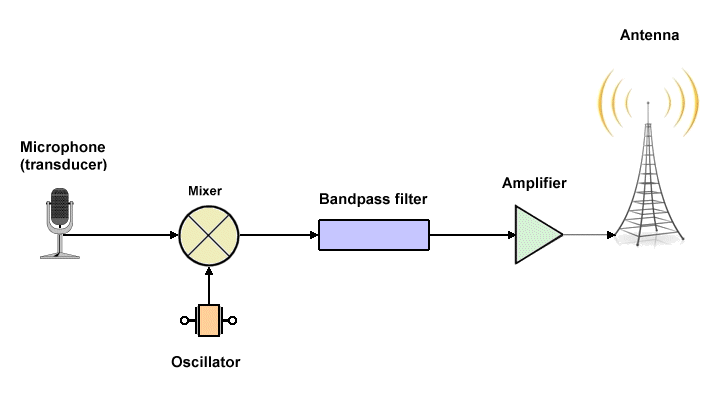A single sideband AM transmitter system

The receiver must restore the carrier signal before demodulation can take place by creating its own carrier signal using a local oscillator and adding it to the received SSB AM signal in a mixer. A suitable receiver system might look something like that shown below.A single sideband AM receiver

The table below lists the ITU designations for various amplitude modulation schemes.

ITU Amplitude Modulation Scheme Designations
DesignationDescription
A3EDouble-sideband full-carrier
R3ESingle-sideband reduced-carrier
H3ESingle-sideband full-carrier
J3ESingle-sideband suppressed-carrier
B8EIndependent-sideband emission
C3FVestigial-sideband
LincompexLinked compressor and expander